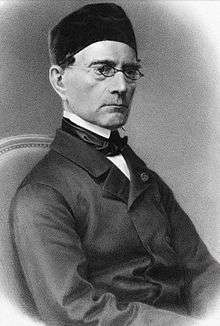# Gabriel Lamé

Gabriel Léon Jean Baptiste LaméBorn 22 July 1795
Tours, France
Died 1 May 1870 (aged 74)
Paris, France
Residence France
Fields Mathematics

Gabriel Léon Jean Baptiste Lamé (22 July 1795 – 1 May 1870) was a French mathematician who contributed to the theory of partial differential equations by the use of curvilinear coordinates, and the mathematical theory of elasticity.

## Biography

Lamé was born in Tours, in today's département of Indre-et-Loire.

He became well known for his general theory of curvilinear coordinates and his notation and study of classes of ellipse-like curves, now known as Lamé curves or superellipses, and defined by the equation:where n is any positive real number.

He is also known for his running time analysis of the Euclidean algorithm, marking the beginning of computational complexity theory. Using Fibonacci numbers, he proved that when finding the greatest common divisor of integers a and b, the algorithm runs in no more than 5k steps, where k is the number of (decimal) digits of b. He also proved a special case of Fermat's last theorem. He actually thought that he found a complete proof for the theorem, but his proof was flawed. The Lamé functions are part of the theory of ellipsoidal harmonics.

He worked on a wide variety of different topics. Often problems in the engineering tasks he undertook led him to study mathematical questions. For example his work on the stability of vaults and on the design of suspension bridges led him to work on elasticity theory. In fact this was not a passing interest, for Lamé made substantial contributions to this topic. Another example is his work on the conduction of heat which led him to his theory of curvilinear coordinates.

Curvilinear coordinates proved a very powerful tool in Lamé's hands. He used them to transform Laplace's equation into ellipsoidal coordinates and so separate the variables and solve the resulting equation.

His most significant contribution to engineering was to accurately define the stresses and capabilities of a press fit joint, such as that seen in a dowel pin in a housing.

In 1854, he was elected a foreign member of the Royal Swedish Academy of Sciences.

Lamé died in Paris in 1870. His name is one of the 72 names inscribed on the Eiffel Tower.